Invest

# Basic Key Figures Of Fundamental Analysis

THE AIM OF FUNDAMENTAL ANALYSIS: REDUCE RISKS!

Could investors have known better? Risks cannot be completely ruled out when investing in stocks, but investors can reduce these risks to a calculable level. The most important tool for valuing stocks is fundamental analysis.

Basic key figures of fundamental analysis

The aim of fundamental analysis is to calculate the “fair value” of a company and its shares. In order to determine this, investment professionals like Winfried Walter check numerous key figures in their fundamental analysis. The basic key figures are included:

• the price-earnings ratio, (P / E)
• the profit yield,
• the dividend yield.

What is the P / E ratio?

The price earnings ratio (PE) is the mother of all key figures. The PER is calculated as follows: price per share divided by earnings per share. An example: Share Y costs 80 dollars. The profit is 5 dollars.

The calculation is:

P / E share Y

80 dollars / 5 dollars = 16 dollars

The P / E ratio is not very meaningful in and of itself. Investors should be careful if the P / E ratio falls or rises sharply from one year to the next. In purely arithmetical terms, the key figure increases if the price picks up while the profit remains the same. Or if the profit collapses at the same rate. Possible causes are:

• prospects for rising profits, which are already priced into the course,
• one-time restructuring costs that are currently affecting profits,
• exploding running costs that permanently weigh on profits.

The indicators also vary from industry to industry and from country to country. Consumer stocks with stable business and strong brand names, for example, are traditionally given a higher P / E ratio than the cyclical automotive industry. In a country comparison, the P / E ratio of US companies is usually higher than that of European companies. However, valuations can also rise because there are few or no investment alternatives. In the current low interest rates, for example, not only the prices of real estate but also stocks have risen on average. If you want to be aware from unpleasant surprises, you should ensure continuity in sales, earnings and cash flow. Outliers then have to be scrutinized very carefully before any judgment can be made about quality and perspectives. A look at the annual report also reveals important information.

When is the P / E ratio meaningful?

The P / E ratio is particularly meaningful in an industry comparison. If two companies in an industry are positioned similarly, the company with the more favorable P / E ratio is preferable. The problem with the ratio: It is based on forecasts.

Because the profit estimated for the calculation is estimated. At the end of 2018, for example, a price-earnings ratio for 2019 is shown, which is based on the average earnings estimates of numerous analysts. It remains to be seen whether the profits will really turn out that way or whether they will be affected by corporate or economic crises.

What is the earnings yield?

The so-called profit yield provides information about the return on capital employed – it is the inverse of the P / E ratio. For the calculation, the earnings per share are set in relation to the price and the result is multiplied by 100%. As in the example above, share Y is quoted at 80 dollars and the profit is 5 dollars. The calculation is then:

Return on earnings share Y:

(5 dollars / 80 dollars) x 100% = 0.0625 x 100% = 6.25%

The earnings yield can be used to compare stocks with one another. But it is also an important benchmark when comparing an equity investment with a bond investment. Earnings yields of 6.25%, as in the completely realistic example, have not been achievable for years with fixed-income securities with good credit ratings .

What is the dividend yield?

Investors also recognize truffles by their dividend yield. The dividend is the portion of the annual profit that shareholders receive by providing equity to the company. The dividend is paid quarterly in some countries. The dividend is similar to the interest paid on federal bonds. However, there is one major difference to fixed income: dividends are only paid if the company also makes a profit. The formula for the calculation: dividend yield divided by the current share price times 100%.

A distribution of 2 dollars for the above-mentioned share results in:

Dividend yield share Y:

(2 dollars / 80 dollars) x 100% = 0.025 x 100% = 2.5%

Many investors make sure that the distributions are constant over the long term and increase as much as possible. On the other hand, caution is advised if the dividend yield fluctuates sharply or shoots up one time. Here the investor may be at the expense of future investments. In contrast, if the share price rises sharply, the dividend yield will decrease. In return, investors are then in the plus thanks to the price gain.•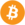Bitcoin (BTC) \$ 20,357.00 1.78%
•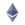Ethereum (ETH) \$ 1,149.99 2.63%
•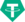Tether (USDT) \$ 1.00 0.04%
•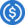USD Coin (USDC) \$ 1.00 0.05%
•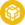BNB (BNB) \$ 228.96 2.04%
•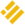Binance USD (BUSD) \$ 1.00 0.01%
•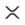XRP (XRP) \$ 0.337345 1.88%
•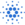Cardano (ADA) \$ 0.473348 1.84%
•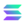Solana (SOL) \$ 35.74 4.7%
•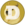Dogecoin (DOGE) \$ 0.067320 3.49%
•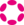Polkadot (DOT) \$ 7.36 2.71%
•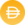Dai (DAI) \$ 1.00 0.08%
•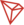TRON (TRX) \$ 0.066117 0.74%
•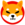Shiba Inu (SHIB) \$ 0.000010 5.99%
•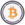Wrapped Bitcoin (WBTC) \$ 20,350.00 1.76%
•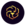LEO Token (LEO) \$ 5.75 1.19%
•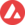Avalanche (AVAX) \$ 18.53 2.63%
•Lido Staked Ether (STETH) \$ 1,103.80 3.21%
•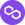Polygon (MATIC) \$ 0.531131 1.78%
•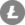Litecoin (LTC) \$ 53.01 2.94%
•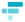FTX (FTT) \$ 25.78 4.68%
•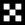OKB (OKB) \$ 12.15 0.72%
•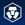Cronos (CRO) \$ 0.120549 2.01%
•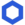Chainlink (LINK) \$ 6.43 0.15%
•Stellar (XLM) \$ 0.115126 0.81%
•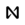NEAR Protocol (NEAR) \$ 3.49 3.17%
•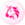Uniswap (UNI) \$ 4.98 4.57%
•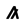Algorand (ALGO) \$ 0.315592 2.48%
•Monero (XMR) \$ 119.15 0.87%
•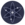Cosmos Hub (ATOM) \$ 7.37 2.88%
•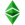Ethereum Classic (ETC) \$ 15.62 3.08%
•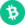Bitcoin Cash (BCH) \$ 105.06 3.71%
•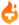Theta Fuel (TFUEL) \$ 0.051742 1.38%
•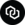Chain (XCN) \$ 0.086161 3.99%
•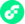Flow (FLOW) \$ 1.68 3.34%
•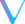VeChain (VET) \$ 0.023673 2.43%
•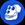ApeCoin (APE) \$ 5.09 4.44%
•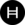Hedera (HBAR) \$ 0.066658 2.55%
•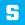The Sandbox (SAND) \$ 1.10 4.29%
•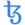Tezos (XTZ) \$ 1.54 3.4%
•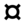Frax (FRAX) \$ 0.992064 0.4%
•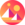Decentraland (MANA) \$ 0.899098 5.41%
•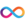Internet Computer (ICP) \$ 5.54 1.75%
•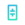Theta Network (THETA) \$ 1.28 2.53%
•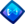Axie Infinity (AXS) \$ 15.45 0.49%
•Filecoin (FIL) \$ 5.56 1.78%
•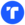TrueUSD (TUSD) \$ 1.00 0.01%
•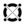Elrond (EGLD) \$ 52.42 4.08%
•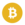Bitcoin SV (BSV) \$ 57.63 1.2%
•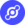Helium (HNT) \$ 9.95 7.85%